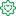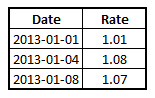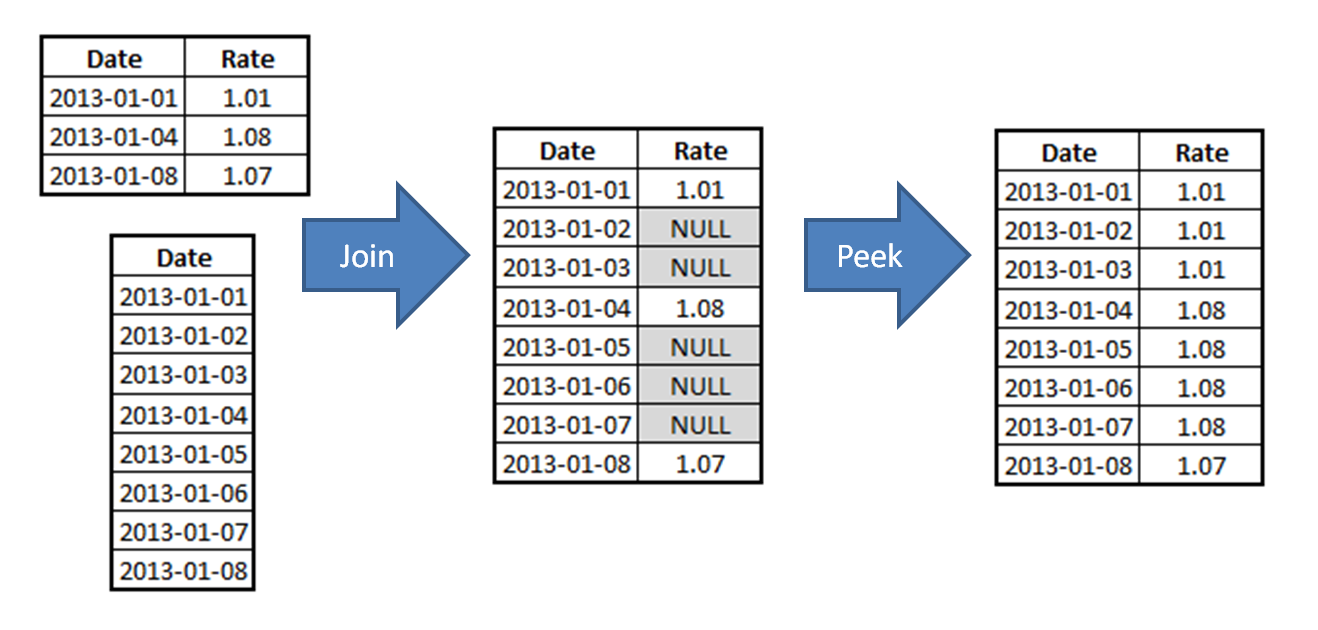Announcements
MAINTENANCE ALERT: Dec. 12th starting 9 AM CET. Community will be read-only. GET DETAILSFormer Employee

## How to populate a sparsely populated field

Sometimes when you load data into QlikView you find that a field is sparsely populated, i.e. it has discrete enumerable values where some values are missing.It could be like in the table to the right where you have three dates, each with some kind of conversion rate. The table only contains the dates where the conversion rate changed, not the dates between the changes.

However, the user will want to ask the question: “What was the status on this specific day?” In other words, the user wants to be able to click on a reference date to see the number that is associated with this date – but the date might not exist in the source data.

In such a situation, you need to generate the missing dates between the changes as individual records and use the “Rate” value from the previous date.

There are several ways to do this in QlikView, and all of them involve some script programming, using temporary tables. One algorithm is

1. Load the source table containing the rates (below called “Rates”).
2. Find largest and smallest date in the “Rates” table.
3. Generate all dates between the largest and smallest dates (below called “Dates”)..
4. Join the “Dates” table (outer join) onto the “Rates” table.
5. Sort the resulting table according to date.
6. Propagate the value of “Rate” downwards to all records that have NULL in the “Rate” field, using the Peek() function.

Visually, the join and peek steps of the algorithm look like this:In the QlikView script, the algorithm would look like the following:

TempTable_Rates:
Load Date, Rate From Rates ;

MinMaxDate:
Load Min (Date ) as MinDate , Max (Date ) as MaxDate resident TempTable_Rates;
Let vMinDate = Peek('MinDate',-1,'MinMaxDate') - 1;
Let vMaxDate = Peek('MaxDate',-1,'MinMaxDate')    ;

Join (TempTable_Rates)

Load Date(recno()+\$(vMinDate)) as Date Autogenerate vMaxDate - vMinDate;

Rates:

If( IsNull( Rate ), Peek( Rate ), Rate ) as Rate
Resident TempTable_Rates
Order By Date ; /* so that above values can be propagated downwards */

Drop Table MinMaxDate, TempTable_Rates;

Problem solved!

This method can be adapted for most situations when you need to insert additional records in existing data: Warehouse balances, Exchange rates, etc.

See more in the Technical Brief: Generating Missing Data In QlikView.

HIC